Author: Oscar Cronquist Article last updated on May 06, 2022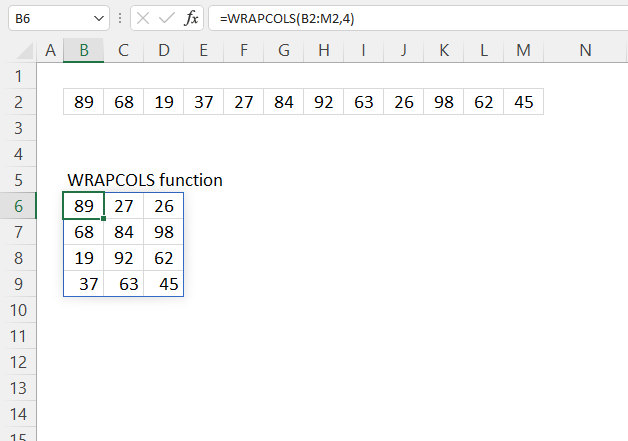The WRAPCOLS function rearranges values from a single row to a 2D cell range based on a given number of values per column.

Dynamic array formula in cell B8:

=WRAPCOLS(B2:M2, 4)

The WRAPCOLS function is available to Excel 365 users and is in the "Array manipulation" category.

## 2. WRAPCOLS Function Arguments

 vector Required. The source cell range or array. wrap_count Required. The number of values per column, the last row may be smaller. [pad_with] Optional. Value to pad with if there is an insufficient number of values in the last row. The default value is #N/A!

## 3. WRAPCOLS Function example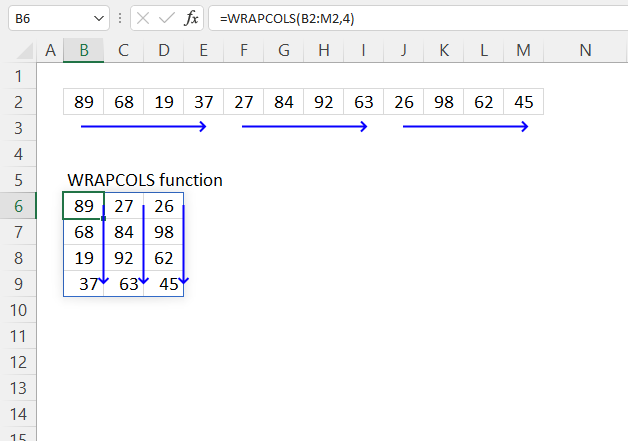The picture above shows how the WRAPCOLS function rearranges values in a row to create a 2D range based on the number of rows specified in the second argument: wrap_count.

Dynamic array formula in cell B8:

=WRAPCOLS(B2:M2, 4)
Note! If any of the values in the source range change, the values in the WRAPCOLS function output change as well.

### 3.1 Explaining formula

vector - B2:M2
wrap_count - 4

#### Step 3 - Evaluate function

WRAPCOLS(B2:M2, 4)

becomes

WRAPCOLS({89, 68, 19, 37, 27, 84, 92, 63, 26, 98, 62, 45})

and returns

{89, 27, 26;
68, 84, 98;
19, 92, 62;
37, 63, 45}.

## 4. WRAPCOLS Function example - pad with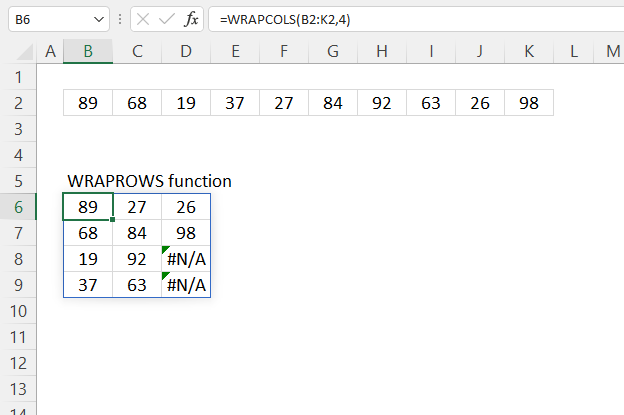#N/A errors show up in empty cells on the last row, use the [pad_with] argument to populate empty cells.

Formula in cell B6:

=WRAPCOLS(B2:K2, 4, "A")

The image below demonstrates how to populate empty cells with the text string "A".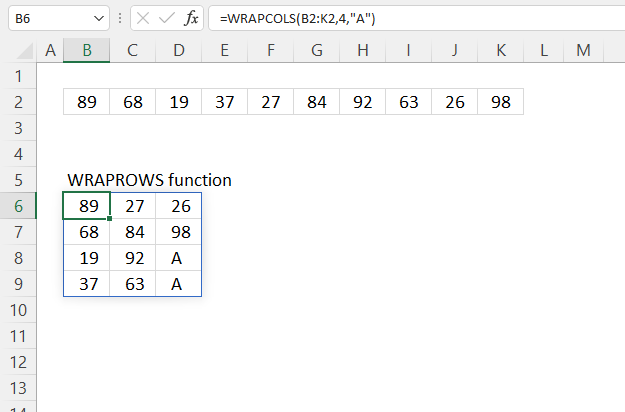Formula in cell B6:

=WRAPCOLS(B2:K2, 4, "A")

## 5. WRAPCOLS function alternative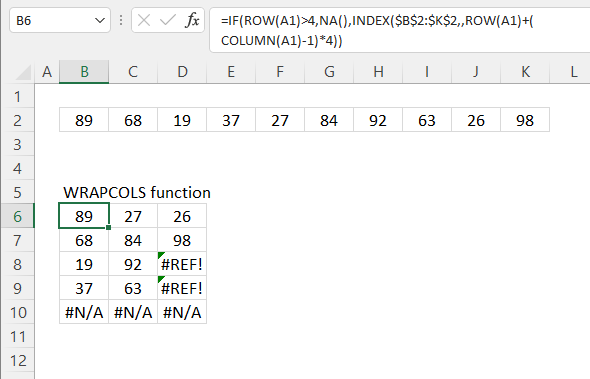This formula wraps the values in cell range B2:K2 to fit four columns. Change the bolded number in the formula below to adjust the number of columns.

Formula in cell B6:

=IF(ROW(A1)>4,NA(),INDEX(\$B\$2:\$K\$2,,ROW(A1)+(COLUMN(A1)-1)*4))

Change bolded numbers to the number of rows you want in the 2D range.

### 5.1 Explaining formula in cell B6

#### Step 1 - Calculate the number of columns in a given cell range

The COLUMN function returns the column number of the top-left cell of a cell reference.

COLUMN(reference)

COLUMN(A1) - 1

becomes

1-1 equals 0 (zero).

#### Step 2 - Calculate column number of relative cell reference

The ROW function returns the row number of a cell reference.

ROW(reference)

ROW(A1)

returns 1.

#### Step 3 - Multiply

The asterisk lets you multiply numbers in an Excel formula.

The parentheses control the order of calculation, we want to subtract before we multiply.

(COLUMN(A1)-1)*4

becomes

0*4 equals 0 (zero).

#### Step 4 - Calculate column number

The INDEX function returns a value from a cell range, you specify which value based on a row and column number.

INDEX(array[row_num][column_num], [area_num])

INDEX(\$B\$2:\$K\$2,,ROW(A1)+(COLUMN(A1)-1)*4)

becomes

INDEX(\$B\$2:\$K\$2,,1)

and returns 89 in cell B6.

#### Step 5 - Return #N/A for row numbers above condition

The IF function returns one value if the logical test is TRUE and another value if the logical test is FALSE.

IF(logical_test, [value_if_true], [value_if_false])

IF(ROW(A1)>4,NA(),INDEX(\$B\$2:\$K\$2,,ROW(A1)+(COLUMN(A1)-1)*4))

becomes

IF(ROW(A1)>4,NA(),89)

becomes

IF(FALSE, NA(), 89)

and returns 89.

## 6. WRAPCOLS function - values in random order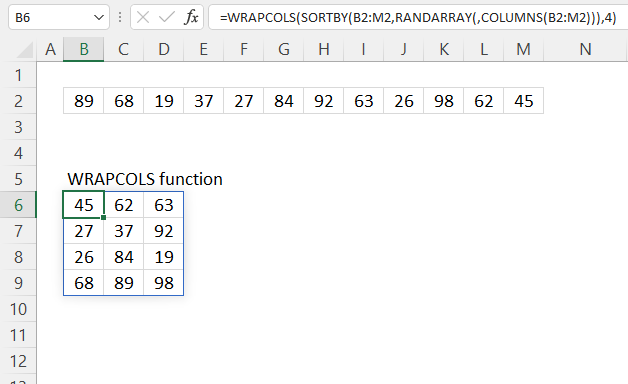The image above shows a formula that rearranges values in random order with four columns.

Dynamic array formula in cell B6:

=WRAPCOLS(SORTBY(B2:M2, RANDARRAY(, COLUMNS(B2:M2))), 4)

### 6.1 Explaining formula in cell B8

#### Step 1 - Calculate the number of cells in cell ref

The COLUMNS function returns the number of columns in a given cell range.

COLUMNS(array)

COLUMNS(B2:M2)

returns 12.

#### Step 2 - Create random decimal values

The RANDARRAY function returns a table of random numbers across rows and columns.

RANDARRAY([rows], [columns], [min], [max], [whole_number])

RANDARRAY(, COLUMNS(B2:M2))

becomes

RANDARRAY(, 12)

and returns

{0.732441255476154, 0.953587479053817, ... , 0.0191675082920368}

#### Step 3 - Sort values based on random decimals

The SORTBY function allows you to sort values from a cell range or array based on a corresponding cell range or array.

SORTBY(array, by_array1, [sort_order1], [by_array2, sort_order2],…)

SORTBY(B2:M2, RANDARRAY(, COLUMNS(B2:M2)))

becomes

SORTBY(B2:M2, {0.732441255476154, 0.953587479053817, ... , 0.0191675082920368})

becomes

SORTBY({89, 68, 19, 37, 27, 84, 92, 63, 26, 98, 62, 45}, {0.732441255476154, 0.953587479053817, ... , 0.0191675082920368})

and returns

{98, 68, 45;27, 19, 26;62, 92, 89;84, 37, 63}.

#### Step 5 - Wrap values

WRAPCOLS(SORTBY(B2:M2, RANDARRAY(, COLUMNS(B2:M2))), 4)

becomes

WRAPCOLS({98, 68, 45;27, 19, 26;62, 92, 89;84, 37, 63}, 4)

and returns

{98, 68, 45;
27, 19, 26;
62, 92, 89;
84, 37, 63}

Press F9 to recalculate values, this creates a new random order.

## 7. WRAPCOLS Function - multiple source ranges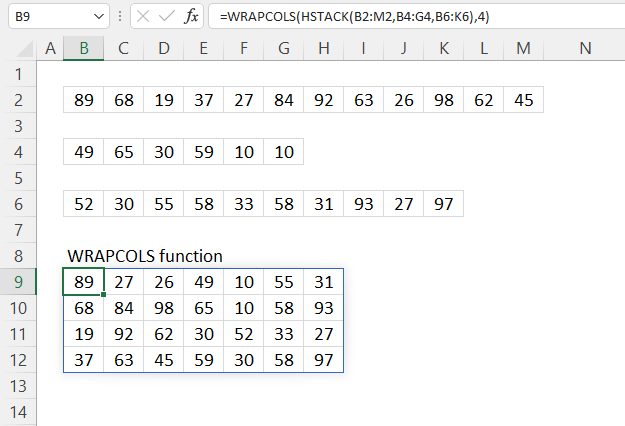The picture above shows a formula that rearranges values in multiple non-adjacent rows to a 2D range.

Dynamic array formula in cell B6:

=WRAPCOLS(HSTACK(B2:M2, B4:G4, B6:K6), 4)

### 8.1 Explaining formula

#### Step 1 - Stack values horizontally

The HSTACK function lets you combine cell ranges or arrays, it joins data to the first blank cell to the right of a cell range or array (horizontal stacking)

HSTACK(array1, [array2],...)

HSTACK(B2:M2, B4:G4, B6:K6)

becomes

HSTACK({89, 68, 19, 37, 27, 84, 92, 63, 26, 98, 62, 45}, {49, 65, 30, 59, 10, 10}, {52, 30, 55, 58, 33, 58, 31, 93, 27, 97})

and returns

{89, 68, 19, 37, 27, 84, 92, 63, 26, 98, 62, 45, 49, 65, 30, 59, 10, 10, 52, 30, 55, 58, 33, 58, 31, 93, 27, 97}.

#### Step 2 - Wrap values to rows

WRAPCOLS(HSTACK(B2:M2, B4:G4, B6:K6), 4)

becomes

WRAPCOLS({89, 68, 19, 37, 27, 84, 92, 63, 26, 98, 62, 45, 49, 65, 30, 59, 10, 10, 52, 30, 55, 58, 33, 58, 31, 93, 27, 97}, 4)

and returns

{89, 27, 26, 49, 10, 55, 31;
68, 84, 98, 65, 10, 58, 93;
19, 92, 62, 30, 52, 33, 27;
37, 63, 45, 59, 30, 58, 97}